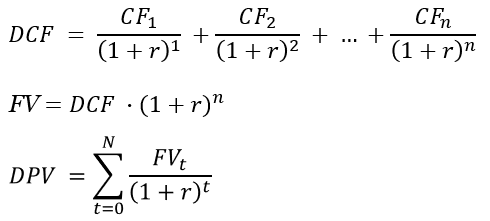# Discounted Cash Flow Calculator

Use this DCF calculator to compute the Discounted Present Value (DPV) of an investment using an analysis based on the Discounted Cash Flow model. Growth phase and terminal phase adjustments included.

\$
%
%
years
%
years
Share calculator:

Embed this tool:
get code

## Using the Discounted Cash Flow calculator

Our online Discounted Cash Flow calculator helps you calculate the Discounted Present Value (a.k.a. intrinsic value) of future cash flows for a business, stock investment, house purchase, etc. Discounted cash flow is more appropriate when future conditions are variable and there are distinct periods of rapid growth and then slow and steady terminal growth.

Start by entering the initial investment, price per share, purchasing price, etc. then enter the discount rate, which is usually the weighted average cost of capital (WACC) for a business valuation, or equivalently the "risk-free rate" for other types of investments, e.g. return from T-bonds. Proceed to enter the expected average growth rate and period over which the investment is expected to experience such growth. Finally, enter the terminal rate and the period over which you expect your investment to increase at significantly reduced rates.

Our DCF calculator will output: Growth Discounted Present Value (a.k.a. growth intrinsic value), Terminal Discounted Present Value (a.k.a. terminal intrinsic value) and the sum of the two values, the Total Discounted Present Value (a.k.a. total intrinsic value). These are the outputs of the DCF model.

## What is Discounted Cash Flow analysis?

It is a method for estimating the business valuation of a project, company or asset based on the time value of money concept, according to which future cash flows are discounted using the cost of capital to arrive at a discounted present value (DPV). Its goal is to estimate the value an investor would receive adjusted by the risk-free rate of an alternative available investment. If the present value estimate is higher than the present-day cost of the investment it makes the project worth considering. As a simple example, consider that \$100 put into a savings account today will be worth \$105 a year from now (5% interest assumed), but if using these \$100 is delayed for a year, it would be worth only \$95 as it could not be put into the savings account.

DCF is useful when one can control and forecast with reasonable accuracy and it sees use in investment finance, real estate development, corporate financial management as well as patent valuation in court cases. As with any other method, garbage in results in garbage out. Among its limitations are the fact that variable discount rate is not supported in its naive form, nor is changing cost of capital.

Another limitation is that the cyclical nature of markets is not accounted for, thus the average return during both the growth stage and terminal stage can be widely inaccurate given significant market fluctuations.

Example application: an investor is considering supporting a company by investing half a million USD for which he will acquire a 5% stake (current company valuation is \$10 million => current value of the 5% stake is \$500,000) and he expects it to grow fast at 15% a year over the next 3 years, and then slow down to about 5% yearly growth for the next 5 years. Is it worth making the investment given that the market average return is expected to be 11% over this period? Plugging the numbers in we see that the total DPV is almost \$4 million, so the \$3 million difference is a good reward for the risk of investing in a single company. However, the difference is only \$600,000 dollars for the first three years, meaning that if a fast exit is of interest he needs to be prepared to get a more modest compensation.

## DCF formula

If you wonder how to calculate the Discounted Present Value (DPV) by yourself or using an Excel spreadsheet, all you need is the formulas for the discounted cash flow (DCF), future value (FV) and, finally, DPV:where r is the discount rate and n is the number of cash flow periods, CF0-n represents the cash flow during each period whereas t is the specific period in the third formula. Σ is the familiar sigma notation used in summation formulas.

## Financial caution

This is a simple online tool which is a good starting point in estimating the Discounted Present Value for any investment, but is by no means the end of such a process. You should always consult a qualified professional when making important financial decisions and long-term agreements, such as long-term bank deposits. Use the information provided by the calculator critically and at your own risk.

#### Cite this calculator & page

If you'd like to cite this online calculator resource and information as provided on the page, you can use the following citation:
Georgiev G.Z., "Discounted Cash Flow Calculator", [online] Available at: https://www.gigacalculator.com/calculators/dcf-calculator.php URL [Accessed Date: 01 Apr, 2023].